# Data-Driven Sparse PLS (ddsPLS)

library(ddsPLS)
#> Le chargement a nécessité le package : foreach
#> Le chargement a nécessité le package : doParallel
#> Le chargement a nécessité le package : iterators
#> Le chargement a nécessité le package : parallel
#> Le chargement a nécessité le package : shiny

## Overview

The objective is to explain a block-matrix $$\mathbf{Y}$$ thanks to a block-matrix $$\mathbf{X}$$. Each block describes $$n$$ observations through $$q$$ numerical variables for the block-matrix $$\mathbf{Y}$$ and $$p$$ numerical variables for the block-matrix $$\mathbf{X}$$. The links are assumed to be linear such as the objective is to estimated a linear matrix transformation $$\mathbf{B}$$ such as

$\mathbf{Y} = \mathbf{X}\mathbf{B} + \mathbf{E},$ where $$\mathbf{E}$$ is an additive random noise.

In the general case, the number of observations $$n$$ can be lower than the number of descriptors $$p$$ and most of regression methods cannot handle the estimation of the matrix $$\mathbf{B}$$, often denoted $$n<\!<p$$. The ddsPLS methodology deals with this framework.

The objective of the proposed method is to estimate the matrix $$\mathbf{B}$$ and to simultaneously select the relevant variables in $$\mathbf{Y}$$ and in $$\mathbf{X}$$.

The methodology gives quality descriptors of the optimal built model, in terms of explained variance and prediction error, and provides a ranking of variable importance.

## Tuning parameters

Only two kinds of parameters need to be tuned in the ddsPLS methodology. This tuning is automatically data driven. The proposed criterion uses the power of bootstrap, a classical statistical tool that generates different samples from an initial one, particularly interesting when the sample size $$n$$ is small.

The tuning parameters are:

• the number $$R$$ of independent links (latent components) between the matrix $$\mathbf{Y}$$ and the matrix $$\mathbf{X}$$.
• For each latent component $$r\in [\![1,R]\!]$$, the regularization coefficient $$\lambda_r\in [0,1]$$, which is easily interpretable as the minimum level of absolute correlation between the variables of $$\mathbf{Y}$$ and $$\mathbf{X}$$ allowed in the building of the current component. For instance, the $$\mathbf{X}$$ selected variables of a built component with $$\lambda_r=0.2$$ are at least correlated to 0.2 with the $$\mathbf{Y}$$ selected variables.

More precisely, the ddsPLS methodology detailed in this vignette is based on $$R$$ soft-thresholded estimations of the covariance matrices between a $$q$$-dimensional response matrix $$\mathbf{Y}$$ and a $$p$$-dimensional covariable matrix $$\mathbf{X}$$. Each soft-threshold parameter is the $$\lambda_r$$ parameter introduced above. It relies on the following latent variable model.

## Quality descriptors

### Construction and prediction errors

The ddsPLS methodology uses the principle of construction and prediction errors. The first one, denoted as $$R^2$$, evaluates the precision of the model on the training data-set. The second one, denoted as $$Q^2$$, evaluates the precision of the model on a test data-set, independent from the train data-set. The $$R^2$$ is well known to be sensible to over-fitting and an accepted rule of thumb, among PLS users, is to select model for which the difference $$R^2-Q^2$$ is minimum.

The ddsPLS methodology is based on bootstrap versions of the $$R^2$$ and the $$Q^2$$ defined below.

### Bootstrap versions of the $$R^2$$ and the $$Q^2$$

More precisely, for the bootstrap sample of index $$b$$, among a total of $$B$$ bootstrap samples, the $$R^2_b$$ and the $$Q^2_b$$ are defined as $\begin{array}{cc} \begin{array}{rccc} &R^{2}_b & = & 1-\dfrac{ \left|\left|\mathbf{y}_{\text{IN}(b)} -\hat{\mathbf{y}}_{\text{IN}(b)}\right|\right|^2 }{ \left|\left|\mathbf{y}_{\text{IN}(b)} -\bar{\mathbf{y}}_{\text{IN}(b)}\right|\right|^2 }, \end{array} & \begin{array}{rccc} &Q^{2}_b & = & 1-\dfrac{ \left|\left|\mathbf{y}_{\text{OOB}(b)} -\hat{\mathbf{y}}_{\text{OOB}(b)}\right|\right|^2 }{ \left|\left|\mathbf{y}_{\text{OOB}(b)} -\bar{\mathbf{y}}_{\text{IN}(b)}\right|\right|^2 }, \end{array} \end{array}$ where the IN(b) (IN for “In Bag”) is the list on indices of the observations selected in the bootstrap sample $$b$$ and OOB(b) (OOB for “Out-Of-Bag”) is the list on indices not selected in the bootstrap sample. Then, the subscripts IN(b) correspond to values of the object taken for in-bag indices (respectively for subscript notation OOB(b) and out-of-bag indices). Also, the “$$\hat{\mathbf{y}}$$” notation corresponds to the estimation of “$$\mathbf{y}$$” by the current model (based on the in-bag sample) and “$$\bar{\mathbf{y}}$$” stands for the mean estimator of “$$\mathbf{y}$$”. The ddsPLS methodology aggregates the $$B$$ descriptors $$R^2_b$$ and $$Q^2_b$$ as follows: $\bar{R}^{2}_{B}=\frac{1}{B}\sum_{b=1}^{B}R^2_b ~~~\mbox{and}~~~ \bar{Q}^{2}_{B}=\frac{1}{B}\sum_{b=1}^{B}Q^2_b.$

Even if the notations of the metrics $$\bar{R}^{2}_{B}$$ and $$\bar{Q}^{2}_{B}$$ show a square, they can be negative. Indeed, for large samples, they actually compare the quality of the built model to the mean prediction model:

• if the metrics is $$>0$$ then the model works better than the mean estimator,
• if the metrics is $$<0$$ then the model works worse than the mean estimator.

As the objective of a linear prediction model is to build models better than the mean prediction model, a rule of thumb is to select models for which $\begin{array}{ccc} \mbox{(A)} &:& \bar{Q}^{2}_{B}>0. \end{array}$

A second rule of thumb is necessary to determine if a new component is relevant to improve the overall prediction power of the model. If the $$r^{th}$$-component is tested, then the condition writes

$\begin{array}{ccc} \mbox{(Ar)} &:& \bar{Q}^{2}_{B,r}>0, \end{array}$

where $$\bar{Q}^{2}_{B,r}$$ is the “component-version” of $$\bar{Q}^{2}_{B}$$” defined in Appendix A.1.

Remark 1 The metrics $$\bar{R}^{2}_{B}$$ and $$\bar{Q}^{2}_{B}$$ are in fact estimators of a same statistic $$\gamma$$, associated with a prediction model $$\mathcal{P}$$: $\begin{array}{ccc} \gamma(\mathcal{P}) & = & 1-\dfrac{ \sum_{j=1}^q \mbox{var} ({y}_{j} -y_{j}^{(\mathcal{P})}) }{ \sum_{j=1}^q \mbox{var} ({y}_{j})}, \end{array}$ where $$y_{j}^{(\mathcal{P})})$$ is the prediction of the $$j^{th}$$ component of $$\mathbf{y}$$ by the model $$\mathcal{P}$$. The closer this statistic is to 1, the more accurate the model $$\mathcal{P}$$ is.

### Automatic tunning of the parameters

As said before, a ddsPLS model of $$r$$ components is based on $$r$$ parameters $$(\lambda_1,\dots, \lambda_r)$$, denoted $$\mathcal{P}_{{\lambda}_1,\dots,{\lambda}_{r}}$$. The metrics $$\bar{R}^{2}_{B}$$, $$\bar{Q}^{2}_{B}$$ or $$\bar{Q}^{2}_{B,r}$$ are functions of the values taken by the estimated ddsPLS models $$\widehat{\mathcal{P}}$$, which depend on the $$r$$ estimated $$\widehat{\lambda}_1,\dots,\widehat{\lambda}_{r}$$ parameter. The ddsPLS methodology is based on a set of to be tested values for estimating each $$\lambda_s$$, $$\forall s\in[\![1,r]\!]$$, which is denoted as $$\Lambda$$ in the following. Their values are pick in $$[0,1]$$.

We denote by $\bar{R}^{2}_{B}(\widehat{\mathcal{P}}_{\widehat{\lambda}_1,\dots,\widehat{\lambda}_{r}})~~~\text{and}~~~\bar{Q}^{2}_{B}(\widehat{\mathcal{P}}_{\widehat{\lambda}_1,\dots,\widehat{\lambda}_{r}})$ the values of the two metrics for the estimated ddsPLS model $$\widehat{\mathcal{P}}$$ of $$r$$ components, based on the $$r$$ estimated regularization coefficients $$\widehat{\lambda}_1,\dots,\widehat{\lambda}_{r}$$. For each component, the ddsPLS methodology seeks the model which minimizes the difference between those two metrics, more precisely:

$\begin{array}{cccc} \widehat{\lambda}_r &=& \mbox{arg min}_{\lambda\in \Lambda} & \bar{R}^{2}_{B}( \widehat{\mathcal{P}}_{\widehat{\lambda}_1,\dots,\widehat{\lambda}_{r-1},\lambda} ) - \bar{Q}^{2}_{B}( \widehat{\mathcal{P}}_{\widehat{\lambda}_1,\dots,\widehat{\lambda}_{r-1},\lambda} ),\\ && \mbox{s.t} & \left\{\begin{array}{l} \bar{Q}^{2}_{B}( \widehat{\mathcal{P}}_{\widehat{\lambda}_1,\dots,\widehat{\lambda}_{r-1},\lambda} )>\bar{Q}^{2}_{B}( \widehat{\mathcal{P}}_{\widehat{\lambda}_1,\dots,\widehat{\lambda}_{r-1}} ),\\ \bar{Q}^{2}_{B,r}( \widehat{\mathcal{P}}_{\widehat{\lambda}_1,\dots,\widehat{\lambda}_{r-1},\lambda} )>0. \end{array}\right. \end{array}$

### Nota bene

The $$r^{th}$$-component is not built if $$\widehat{\lambda}_r =\emptyset$$ and so the selected model is a $$(r-1)$$-component (if $$r-1>0$$ or is the mean estimator model) with estimated values for the regularization coefficients $$(\widehat{\lambda}_1,\dots,\widehat{\lambda}_{r-1})$$.

The package depends on a low number of low level packages. They are of two types:

• Parallelization packages: foreach, parallel and doParallel (which depends on both of the previous packages).

• $$\mathbf{C}^{++}$$-developer packages: Rcpp (for basic $$\mathbf{C}^{++}$$ development) and RcppEigen (for inner mathematical operations).

devtools::install_github("hlorenzo/ddsPLS")
library(ddsPLS)

## A Latent Variable Model

In the following, we study a synthetic structure defined as, in general

$\left\{ \begin{array}{l} \mathbf{x} = \mathbf{A}'\boldsymbol{\phi} + \boldsymbol{\epsilon},\ \mathbf{A}\in\mathbb{R}^{R\times p}, \\ \mathbf{y} = \mathbf{D}'\boldsymbol{\phi}+ \boldsymbol{\xi},\ \mathbf{D}\in\mathbb{R}^{R\times q}, \end{array} \right.$

where $$R$$ is the total number of eigenvectors of $$\mathbf{A}'\mathbf{A}=\mbox{var}\left(\mathbf{x}\right)$$ with non-null projections on $$\mathbf{A}'\mathbf{D}=\mbox{cov}\left(\mathbf{x},\mathbf{y}\right)$$. In the PLS context this is the theoretical number of components.

### A high-dimensional structure

For the sack of the proposed simulations, we use $$R=2$$ (associated to the dimension of $$\boldsymbol{\phi}$$), $$p=1000$$ (associated to the dimension of $$\mathbf{A}'\boldsymbol{\phi}$$ and $$\boldsymbol{\epsilon}$$) and $$q=3$$ (associated to the dimension of $$\mathbf{D}'\boldsymbol{\phi}$$ and $$\boldsymbol{\xi}$$).

The total number of such data-sets is rarely higher than $$p=1000$$ and the constraint $$n<\!<p$$ holds most of times. This is an high-dimensionnal data-set.

Each observation of those random vectors are generated following a multivariate normal distribution such as $\boldsymbol{\psi}=(\boldsymbol{\phi}',\boldsymbol{\epsilon}_{1,\dots,100}'/\sigma,\boldsymbol{\epsilon}_{101,\dots,1000}',\boldsymbol{\xi}_{1,2}',\xi_3)'\sim \mathcal{N}\left(\mathbf{0}_{2+1000+3},\mathbb{I}_{2+1000+3}\right),$

where $$\sigma$$ is the standard deviation of the additive noise. A response variable $$\mathbf{y}$$ of $$q=3$$ components is generated as a linear combination of the latent variable $$\boldsymbol{\phi}$$ to which is added a Gaussian noise $$\boldsymbol{\xi}$$. The equivalent process generates a variable $$\mathbf{x}$$ of $$p=1000$$ components, from the matrix $$\mathbf{A}$$ and Gaussian additive noise $${\sigma}=\sqrt{1-0.95^2}\approx0.312$$. The columns of $$\mathbf{A}$$ and $$\mathbf{D}$$ are normalized such as

$\forall(i,j)\in[\![1,p]\!]\times[\![1,q]\!],\ \mbox{var}({x}_i)=\mbox{var}({y}_j)=1.$

Remark 2 Taking into account Remark 1 and the current statistical model, we can define a theoretical (understanding if $$n\rightarrow +\infty$$) value for $$\gamma(\mathcal{P})$$ which is $\begin{array}{ccc} \gamma^\star & = & 1-\dfrac{ \sum_{j=1}^q \mbox{var} (\epsilon_j) }{ \sum_{j=1}^q \mbox{var} ({y}_{j})}= 2(1-\sigma^2)/3\approx0.602. \end{array}$ Comparing this theorethical value to the values of $$\bar{Q}^2_B$$ helps interpretability, for theorethical work only. If $$\bar{Q}^2_B<\!<\gamma^\star$$ then the corresponding model is not enough efficient. If $$\bar{Q}^2_B>\!>\gamma^\star$$ then the corresponding model overfits the data.

### Correlation structure

More precisely we propose to study the following matrices $$\mathbf{A}$$ and $$\mathbf{D}$$ $\begin{array}{c l c c} & \mathbf{A} =\sqrt{1-\sigma^2} \left(\begin{array}{ccc} \boldsymbol{1}_{50}' & \sqrt{\alpha}\boldsymbol{1}_{25}' & \boldsymbol{0}_{25}' & \boldsymbol{0}_{900}'\\ \boldsymbol{0}_{50}' & \sqrt{1-\alpha}\boldsymbol{1}_{25}' & \boldsymbol{0}_{25}' & \boldsymbol{0}_{900}'\\ \boldsymbol{0}_{50}' & \boldsymbol{0}_{25}' &\boldsymbol{1}_{25}' & \boldsymbol{0}_{900}'\\ \end{array} \right)_{(3,1000)} &\text{ and }& \mathbf{D}=\sqrt{1-\sigma^2} \left(\begin{array}{ccc} 1 & \sqrt{\beta_0} & 0 \\ 0 & \sqrt{1-\beta_0} & 0 \\ 0 & 0 & 0 \\ \end{array} \right)_{(3,3)}, \end{array}$ where $$\alpha\in [0,1]$$ can be easily interpreted. Indeed, $$\alpha$$ controls the correlation between the components $$\mathbf{x}_{1\dots 50}$$ and $$\mathbf{x}_{51\dots 75}$$. Also $$\beta_0=0.1$$. It indirectly controls the association between $$\mathbf{x}$$ and $$y_2$$. The effects of $$\alpha$$ on those two associations are detailed in the following table.

Value of $$\alpha$$ $$\alpha\approx 0$$ $$\alpha\approx 1$$
$$\mbox{cor}(\mathbf{x}_{1\dots 50},\mathbf{x}_{51\dots 75})$$ Strong Low
$$\mbox{cor}(\mathbf{x},y_2)$$ Strong Low

If $$\alpha> 1/2$$, the second variable response component, $$y_2$$, is hard to predict and the variables $$\mathbf{x}_{51\dots 75}$$ are hard to select. The objective is to build models predicting $$y_1$$ and $$y_2$$ but not $$y_3$$. Also components 1 to 75 of $$\mathbf{x}$$ should be selected.

The function getData, printed in Appendix A.2 allows to simulate a couple (X,Y) according to this structure.

## A low correlated data-set

We assume that $$\alpha=0.1$$ and we simulate the data thanks to the function given in Appendix A.2. For this simulation, a sample of $$n=200$$ observations is chosen

eps <- 0.95
n <- 100
alpha <- 0.1
datas <- getData(n=n,alpha=alpha,beta_0=0.1,sigma=sqrt(1-eps^2),
p1=50,p2=25,p3=25,p=300)

It is also a necessity to fix the grid of $$\lambda$$, denoted as $$\Lambda$$ in the previous section and lambdas in the following. Also the number n_B of bootstrap samples is chosen, fixed to 20.

lambdas <- seq(0,1,length.out = 30)
n_B <- 20

Finally we have chosen to build the model working on NCORES=4 CPUs.

NCORES <- 4
mo <- ddsPLS( datas$X, datas$Y,lambdas = lambdas,
n_B=n_B,NCORES=NCORES,verbose = F)

Once the model is built, it is easy to check its performance thanks to the summary S3-method.

sum_up <- summary(mo,return = T)
#>                       ______________
#>                      |    ddsPLS    |
#> =====================----------------=====================
#> The optimal ddsPLS model is built on 2 component(s)
#>
#> The bootstrap quality statistics:
#> ---------------------------------
#>         lambda   R2 R2_r   Q2 Q2_r
#> Comp. 1   0.52 0.34 0.34 0.32 0.32
#> Comp. 2   0.52 0.58 0.24 0.57 0.36
#>
#>
#> The explained variance (in %):
#> -----------------------
#>
#> In total: 58.37
#> -  -  -
#>
#> Per component or cumulated:
#> -  -  -  -  -  -  -  -  -
#>                Comp. 1 Comp. 2
#> Per component    34.34   24.03
#> Cumulative       34.34   58.37
#>
#> Per response variable:
#> -  -  -  -  -  -  -  -
#>              Y1    Y2 Y3
#> All_comps 90.43 84.68  0
#>
#> Per response variable per component:
#> -  -  -  -  -  -  -  -  -  -  -  -
#>            Y1    Y2 Y3
#> Comp. 1 90.43 12.58  0
#> Comp. 2  0.00 72.09  0
#>
#> ...and cumulated to:
#> -  -  -  -  -  -  -
#>            Y1    Y2 Y3
#> Comp. 1 90.43 12.58  0
#> Comp. 2 90.43 84.68  0
#>
#> For the X block:
#> -  -  -  -  -  -  -  -  -
#>                Comp. 1 Comp. 2
#> Per component    15.07    6.60
#> Cumulative       15.07   21.67
#> =====================                =====================
#>                      ================

A $$2$$-components model has been built and different metrics are detailed. We can discuss different points:

• the estimated values for $$\lambda_1$$ and $$\lambda_2$$ are 0.41 and 0.55 which means that no correlation between any X or Y variables lower than 0.41 will be considered for building the components and so for building the regression matrix.
• the variable $$\mathbf{Y}_3$$ is not selected.
• the metrics of prediction error is equal to $$\bar{Q}_{B}^2=0.58$$ which is below the theoretical value $$\gamma^\star\approx0.602$$.

Also, this S3-method can be used to get the list of the selected variables (and the figure of the Yes/No selction variable, as visible). The high dimension of the data does not allow to conclude directly, the following command allows to say that the variables selected in the X data set are exactly the one desired (since the two lists are exactly the same).

setdiff(1:75,mo$Selection$X)
#> integer(0)

## Evaluate the quality of the model

### Compare with “non selection” ddsPLS model

As a popint of comparison, we can build the ddsPLS model for which $$\Lambda=\{0\}$$ and look at its prediction performances, through the $$\bar{Q}_B^2$$ statistics.

mo0 <- ddsPLS( datas$X, datas$Y,lambdas = 0,
n_B=n_B,NCORES=NCORES,verbose = F)
sum0 <- summary(mo0,return = T)
print(sum0$R2Q2[,c(1,4)]) mo0 <- ddsPLS( datas$X, datas$Y,lambdas = 0, n_B=n_B,NCORES=1,verbose = F) sum0 <- summary(mo0,return = T) #> ______________ #> | ddsPLS | #> =====================----------------===================== #> The optimal ddsPLS model is built on 2 component(s) #> #> The bootstrap quality statistics: #> --------------------------------- #> lambda R2 R2_r Q2 Q2_r #> Comp. 1 0 0.40 0.40 0.35 0.35 #> Comp. 2 0 0.62 0.21 0.53 0.28 #> #> #> The explained variance (in %): #> ----------------------- #> #> In total: 60.28 #> - - - #> #> Per component or cumulated: #> - - - - - - - - - #> Comp. 1 Comp. 2 #> Per component 40.07 20.21 #> Cumulative 40.07 60.28 #> #> Per response variable: #> - - - - - - - - #> Y1 Y2 Y3 #> All_comps 90.87 86.08 3.89 #> #> Per response variable per component: #> - - - - - - - - - - - - #> Y1 Y2 Y3 #> Comp. 1 83.06 37.07 0.08 #> Comp. 2 7.81 49.02 3.81 #> #> ...and cumulated to: #> - - - - - - - #> Y1 Y2 Y3 #> Comp. 1 83.06 37.07 0.08 #> Comp. 2 90.87 86.08 3.89 #> #> For the X block: #> - - - - - - - - - #> Comp. 1 Comp. 2 #> Per component 17.2 6.84 #> Cumulative 17.2 24.04 #> ===================== ===================== #> ================ It is possible to compare the prediction qualities of the two models using print(sum0$R2Q2[,c(1,4)])
#>         lambda        Q2
#> Comp. 1      0 0.3490421
#> Comp. 2      0 0.5335431
print(sum_up\$R2Q2[,c(1,4)])
#>            lambda        Q2
#> Comp. 1 0.5172414 0.3247571
#> Comp. 2 0.5172414 0.5675409

In that context, the sparse ddsPLS approach allows to get better prediction rate than the “non selection” ddsPLS model.

### The S3-methodplot

It is also possible to plot different things thanks to the plot S3-method. In the representation with $$\lambda$$ in abscissa:

• the $$\bullet$$ points correspond to $$\lambda$$ values for which the constraint of the optimization problem detailed in the section Automatic tunning of the parameters are active.
• the large $$\circ$$ points corresponds to the selected value.

The different values given to the argument type would give representation that helps the analyst concluding on the final quality of the model. The different values are

• type="predict" to draw the predicted values of y against the observed. This can be useful to locate potential outliers (observations away from the distribution…) that would drive the model (… but close to the bisector).
• type="criterion" to draw the values of the metrics $$\bar{R}_{B}^2-\bar{Q}_{B}^2$$. This is the optimized metrics.
• type="Q2" to draw the $$\bar{Q}_{B}^2$$ metrics which represents the overall prediction quality of the build model, one component after another.
• type="prop" to draw the proportion of bootstrap models with a positive $$Q_{b,r}^2$$, this $$\forall b \in [\![1,B]\!]$$. Since this is tricky to interpret negative values for $$Q_{b,r}^2$$ (apart from describing models which perform worse than the mean prediction model) negative values for $$\bar{Q}_{B,r}^2$$ is necessarily hardly interpretable. However, the proportion of models with positive $$Q_{b,r}^2$$ can be interpreted as a probability to finally build a model $$\mathcal{P}$$ with a positive $$\gamma(\mathcal{P})$$. This can be interesting to look at this metrics to interpret
• type="weightX" or type="weightY" to draw the values of the weights for each component for the X block of for the Y block. If there is an a priori, such as a functional one, on the variables of X (resp. Y), this a priori (which is not currently taken into account in the ddsPLS model) must certainly have an impact on the values of the weights parameters. This can be characterized by a structure of the weights on each component. In the opposite case, if the model is not enough sparse or too sparse, for example, the analyst is invited to modify the parameterization of the model, by limiting the grid of accessible $$\lambda$$ for example.

### Predicted versus observed values of the response

Simply specifying type="predict".

plot(mo,type="predict",legend.position = "topleft")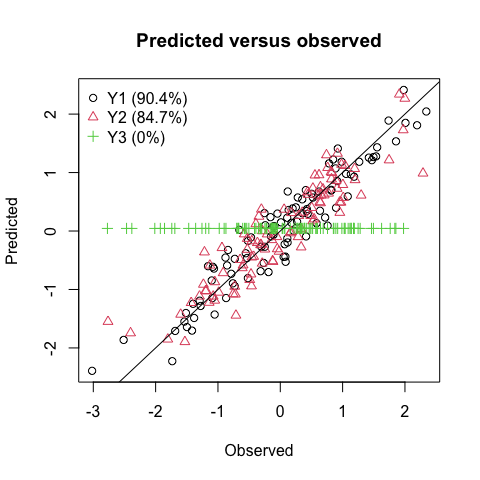Since the the variable $$y_3$$ has not been selected, its predicted values are constantly equal to the mean estimation. The two other columns of Y are described with more than 90% accuracy and no observation seems to guide the model at the expense of other observations.

### The criterion $$\bar{R}_{B}^2-\bar{Q}_{B}^2$$

To plot the criterion, the plot argument type must be set to criterion. It is possible to move the legend with the legend.position argument.

plot(mo,type="criterion",legend.position = "top")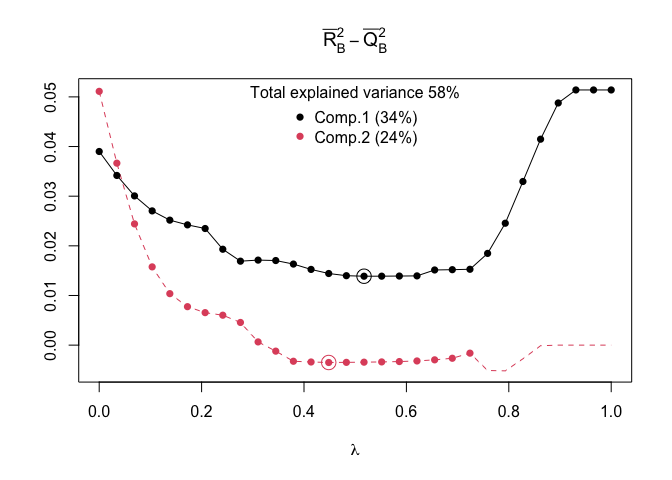The legend title gives the total explained variance by the model built on the two components while the legend itself gives the explained variance by each of the considered component.

### The $$\bar{Q}_{B}^2$$ metrics

the previous figure does not provide information on the prediction quality of the model. This information can be found using the same S3-method fixing the parameter type to type="Q2".

plot(mo,type="Q2",legend.position = "bottomleft")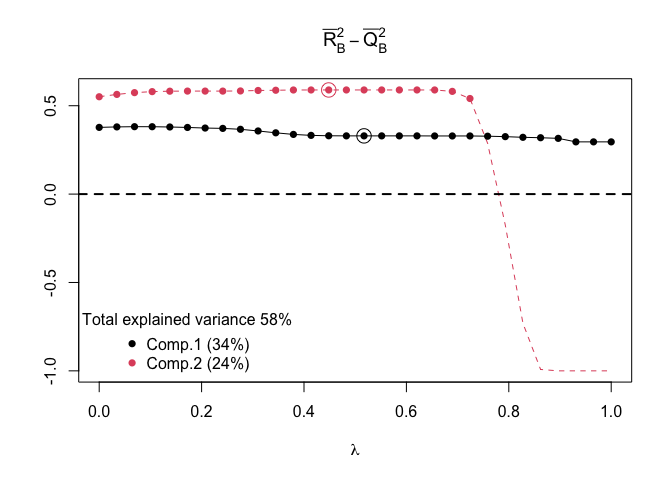According to that figure it is clear that the chosen optimal model (minimizing $$\bar{R}_{B}^2-\bar{Q}_{B}^2$$) represents a model for which the $$\bar{Q}_{B}^2$$ on each of its component is not far from being maximum.

### The proportion of informational bootstrap models

plot(mo,type="prop",legend.position = "bottomleft")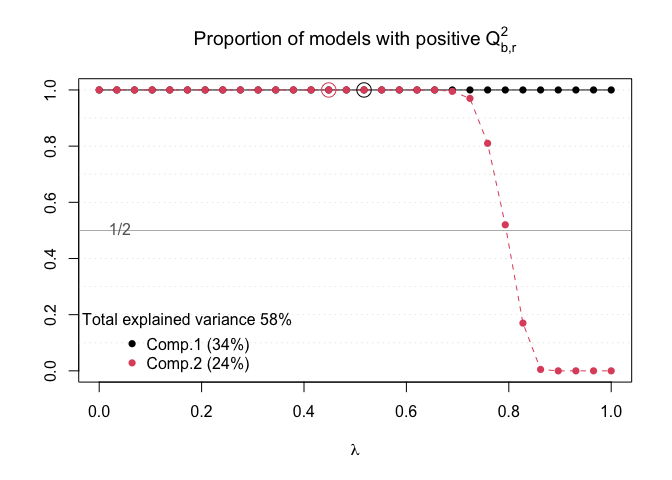#### Plot of the weights

It is also possible to directly plot the values of the weights for each component. For the Y block and for the X block, such as

plot(mo,type="weightY",mar=c(4,7,2,1))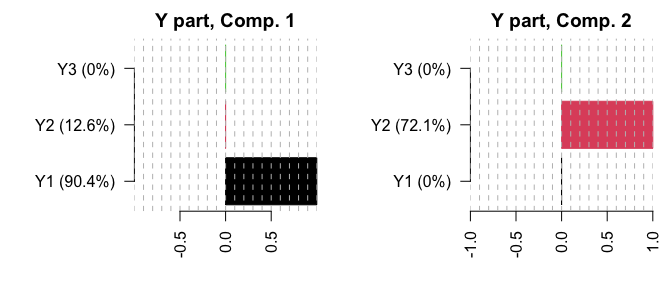where it is clear that the first component explains $$y_1$$ while the second one explains $$y_2$$. Looking at the weights on the block X:

plot(mo,type="weightX",cex.names = 0.5 )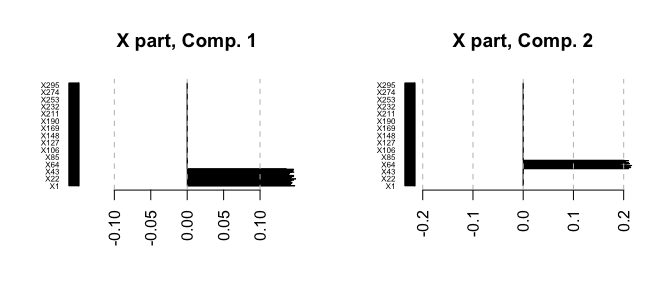the variables $$\mathbf{x}_{1\dots 50}$$ (resp. $$\mathbf{x}_{51\dots 75}$$) are selected on the first (resp. second) component, which is associated only with $$y_1$$ (resp. $$y_2$$).

## Appendix

### A.1 Definition of the “component-$$r$$$$\bar{Q}_B^2$$” denoted $$\bar{Q}_{B,r}^2$$

For a given bootstrap sample indexed by $$b$$, we define $\begin{array}{rccc} &Q^{2}_{b,r} & = & 1-\dfrac{ \left|\left|\mathbf{y}_{\text{OOB}(b)} -\hat{\mathbf{y}}_{\text{OOB}(b)}^{(r)}\right|\right|^2 }{ \left|\left|\mathbf{y}_{\text{OOB}(b)} -\bar{\mathbf{y}}_{\text{IN}(b)}^{(r-1)}\right|\right|^2 }, \end{array}$

where, $$\hat{\mathbf{y}}_{\text{OOB}(b)}^{(r)}$$ is the prediction of $${\mathbf{y}}_{\text{OOB}(b)}$$ for the model built on $$r$$ components. The interpretation of this metrics is that if $$Q^{2}_{b,r}>0$$ then the $$r$$-components based model predicts better than the $$r-1$$-components based model. Naturally, the aggregated version of this metrics is

$\bar{Q}^{2}_{B,r}=\frac{1}{B}\sum_{b=1}^{B}Q^2_{b,r}$

### A.2 Function to simulate the high-dimensional data sets

We propose the following function

getData
#> function(n=100,alpha=0.4,beta_0=0.2,sigma=0.3,
#>                     p1=50,p2=25,p3=25,p=1000){
#>   R1 = R2 = R3 <- 1
#>   d <- R1+R2+R3
#>   A0 <- matrix(c(
#>     c(rep(1/sqrt(R1),p1),rep(sqrt(alpha),p2),rep(0,p3),rep(0,p-p1-p2-p3)),
#>     c(rep(0,p1),rep(sqrt(1-alpha),p2),rep(0,p3),rep(0,p-p1-p2-p3)),
#>     c(rep(0,p1),rep(0,p2),rep(1,p3),rep(0,p-p1-p2-p3))
#>   ),nrow = d,byrow = T)
#>   A <- eps*A0
#>   D0 <- matrix(c(1,0,0,
#>                  sqrt(beta_0),sqrt(1-beta_0),0,
#>                  0,0,0),nrow = d,byrow = F)
#>   D <- eps*D0
#>   q <- ncol(D)
#>   L_total <- q+p
#>   psi <- matrix(rnorm((d+L_total)*n,mean = 0,1),n)
#>   phi <- psi[,1:d,drop=F]
#>   errorX <- matrix(rep(sqrt(1-apply(A^2,2,sum)),n),n,byrow = T)
#>   errorY <- matrix(rep(sqrt(1-apply(D^2,2,sum)),n),n,byrow = T)
#>   X <- phi%*%A + errorX*psi[,d+1:p,drop=F]
#>   Y <- phi%*%D + errorY*psi[,d+p+1:q,drop=F]
#>   list(X=X,Y=Y)
#> }
#> <bytecode: 0x7fd035ed2e50>

where the output is a list of two matrices X and Y.

### A.3 Definitions of the explained variances…

#### A.3.1 Cumulated

For a model built on $$R$$ components

$\begin{array}{rccc} &\text{ExpVar}_{1:R} & = & \left(1-\frac{1}{n}\sum_{i=1}^n\dfrac{ \left|\left|\mathbf{y}_i -\hat{\mathbf{y}}^{(1:R)}_i\right|\right|^2 }{ \left|\left|\mathbf{y}_i -\boldsymbol{\mu}_{\mathbf{y}}\right|\right|^2 } \right)*100, \end{array}$

where

$\hat{\mathbf{y}}^{(1:R)} = \left( \mathbf{X}-\boldsymbol{\mu}_{\mathbf{x}} \right) \mathbf{U}_{(1:R)}\left(\mathbf{P}_{(1:R)}'\mathbf{U}_{(1:R)}\right)^{-1}\mathbf{C}_{(1:R)} + \boldsymbol{\mu}_{\mathbf{y}},$ and $$\mathbf{U}_{(1:R)}$$ is the concatenation of the $$R$$ weights, $$\mathbf{P}_{(1:R)}$$ is the concatenation of the $$R$$ scores $$\mathbf{p}_r=\mathbf{X}^{(r)'}\mathbf{t}_r/\mathbf{t}_r'\mathbf{t}_r$$ and $$\mathbf{C}_{(1:R)}$$ is the concatenation of the $$R$$ scores $$\mathbf{c}_r=\boldsymbol{\Pi}_r\mathbf{Y}^{(r)'}\mathbf{t}_r/\mathbf{t}_r'\mathbf{t}_r$$. The deflated matrices are defined such as $\mathbf{X}{^{(r+1)}} =\mathbf{X}^{(r)}-\mathbf{t}_r\mathbf{p}_r',\ \mathbf{Y}{^{(r+1)}} = \mathbf{Y}{^{(r)}} - \mathbf{t}_r\mathbf{c}_r',$ and $$\boldsymbol{\Pi}_r$$ is the diagonal matrix with 1 element if the associated response variable is selected and 0 elsewhere. Also, $$\boldsymbol{\mu}_{\mathbf{y}}$$ and $$\boldsymbol{\mu}_{\mathbf{x}}$$ are the estimated mean matrices.

##### A.3.2 Per component

$\begin{array}{rccc} &\text{ExpVar}_{R} & = & \left(1-\frac{1}{n}\sum_{i=1}^n\dfrac{ \left|\left|\mathbf{y}_i -\hat{\mathbf{y}}^{(R)}_i\right|\right|^2 }{ \left|\left|\mathbf{y}_i -\boldsymbol{\mu}_{\mathbf{y}}\right|\right|^2 } \right)*100, \end{array}$

##### A.3.3 Per response variable

$\begin{array}{rccc} &\text{ExpVar}_{1:R}^{(j)} & = & \left( 1-\frac{1}{n}\sum_{i=1}^n\dfrac{ \left|\left|y_{i,j} -\hat{{y}}^{(1:R)}_{i,j}\right|\right|^2 }{ \left|\left|{y}_{i,j} -{\mu}_{{y}_j}\right|\right|^2 } \right)*100, \end{array}$

##### A.3.4 Per response variable per component

$\begin{array}{rccc} &\text{ExpVar}_{R}^{(j)} & = & \left( 1-\frac{1}{n}\sum_{i=1}^n\dfrac{ \left|\left|y_{i,j} -\hat{{y}}^{(R)}_{i,j}\right|\right|^2 }{ \left|\left|{y}_{i,j} -{\mu}_{{y}_j}\right|\right|^2 } \right)*100, \end{array}$